## Method

Lidar Odometry and Mapping [la] [LOAM]

Submitted on 25 Mar. 2020 21:03 by
Ji Zhang (Carnegie Mellon University)

 Running time: 0.1 s Environment: 2 cores @ 2.5 Ghz (C/C++)

 Method Description: Scan matching based odometry using Velodyne data Research videos are available at: www.youtube.com/c/JiZhang_CMU Latest, improved method is commercialized by: www.kaarta.com Full-length paper: J. Zhang and S. Singh. Laser-visual-inertial Odometry and Mapping with High Robustness and Low Drift. Journal of Field Robotics. vol. 35, no. 8, pp. 1242–1264, 2018. Parameters: Point to line/plane scan matching Latex Bibtex: @inproceedings{Zhang2014RSS, title = {{LOAM}: Lidar Odometry and Mapping in Real- time}, author = {Ji Zhang and Sanjiv Singh}, booktitle = {Robotics: Science and Systems Conference (RSS)}, month = {July}, year={2014}, address={Berkeley, CA} }

## Detailed Results

From all test sequences (sequences 11-21), our benchmark computes translational and rotational errors for all possible subsequences of length (5,10,50,100,150,...,400) meters. Our evaluation ranks methods according to the average of those values, where errors are measured in percent (for translation) and in degrees per meter (for rotation). Details for different trajectory lengths and driving speeds can be found in the plots underneath. Furthermore, the first 5 test trajectories and error plots are shown below.

## Test Set Average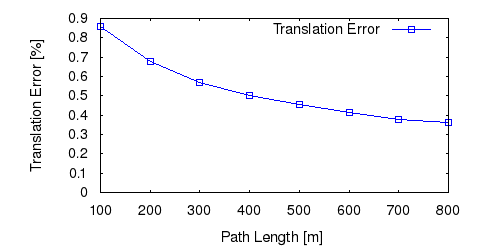This figure as: png eps pdf txt gnuplot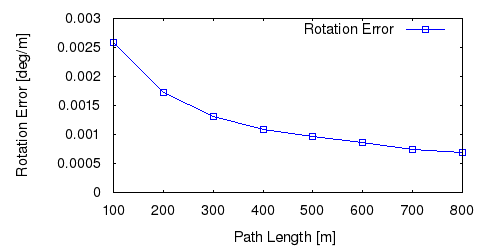This figure as: png eps pdf txt gnuplot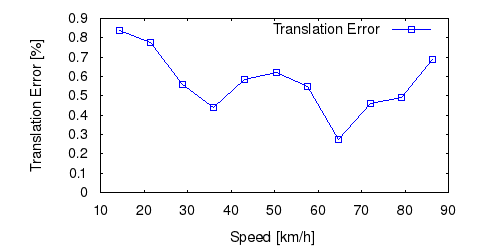This figure as: png eps pdf txt gnuplot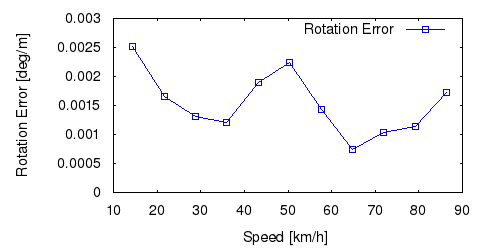This figure as: png eps pdf txt gnuplot

## Sequence 11This figure as: png eps pdf txt gnuplot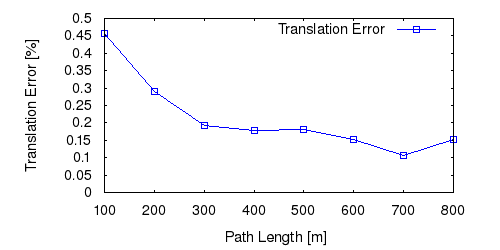This figure as: png eps pdf txt gnuplot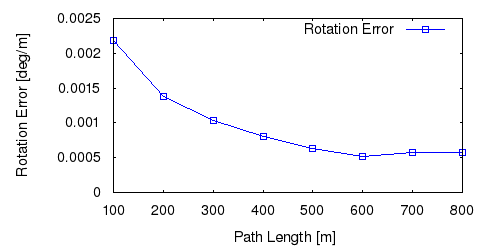This figure as: png eps pdf txt gnuplot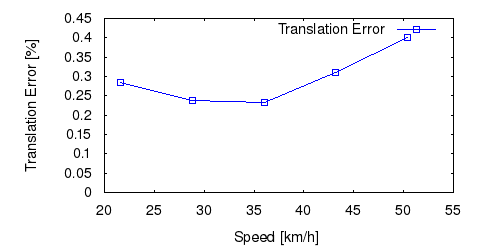This figure as: png eps pdf txt gnuplot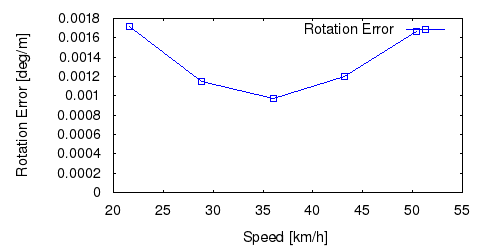This figure as: png eps pdf txt gnuplot

## Sequence 12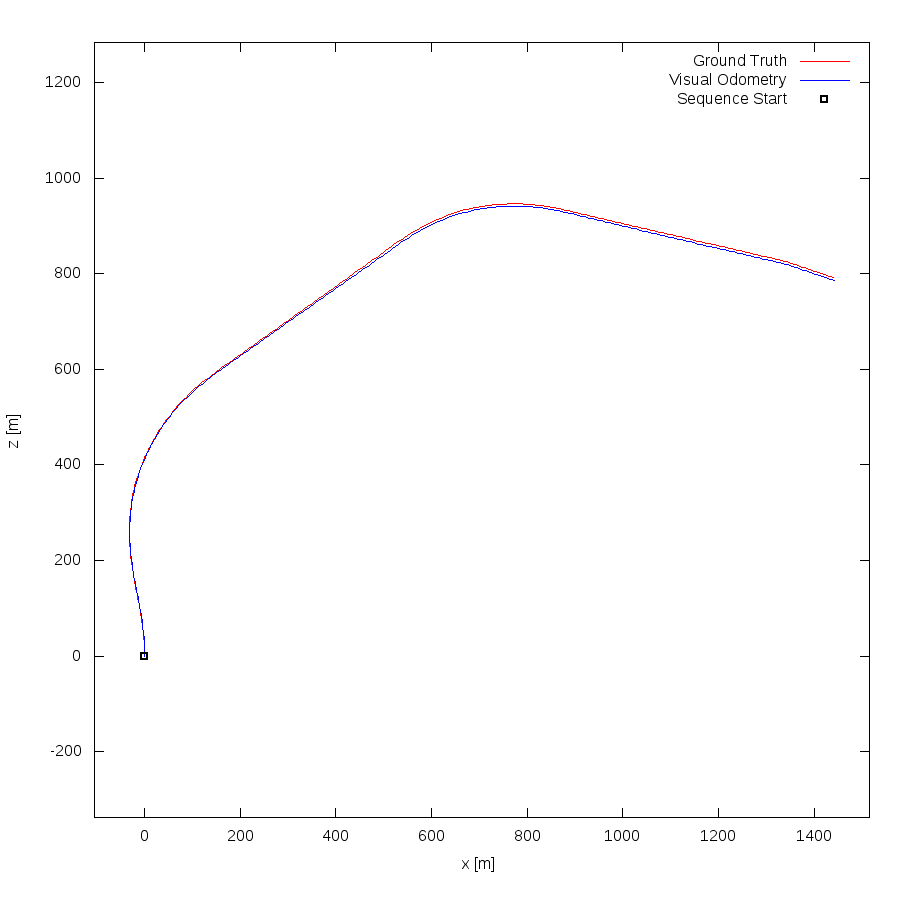This figure as: png eps pdf txt gnuplot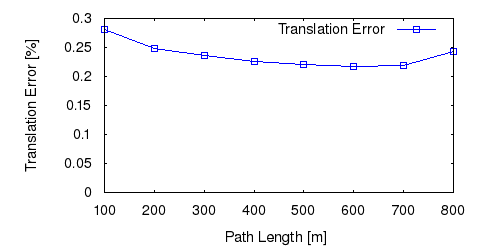This figure as: png eps pdf txt gnuplot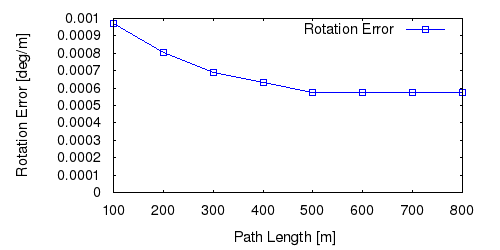This figure as: png eps pdf txt gnuplot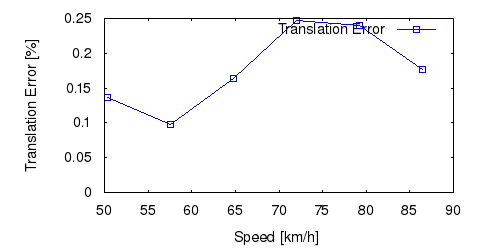This figure as: png eps pdf txt gnuplot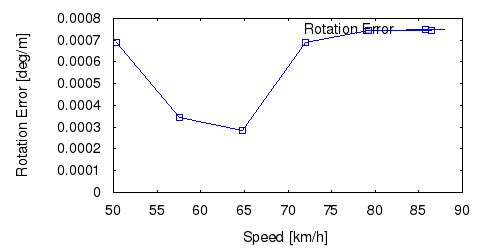This figure as: png eps pdf txt gnuplot

## Sequence 13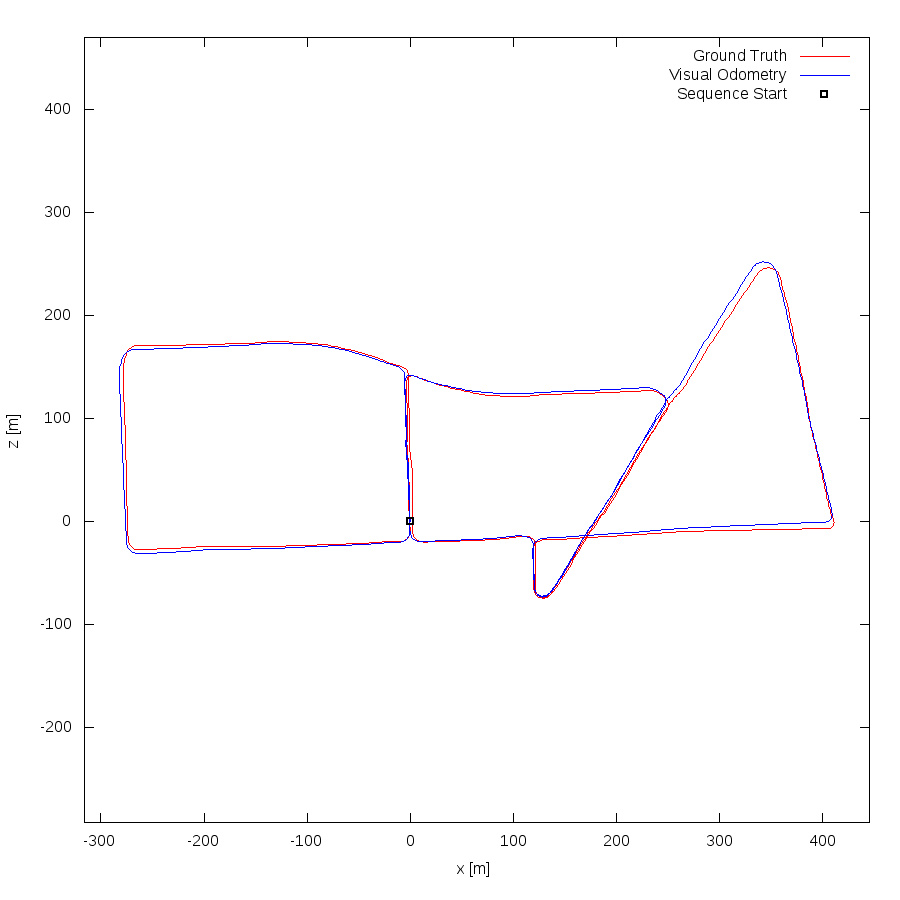This figure as: png eps pdf txt gnuplot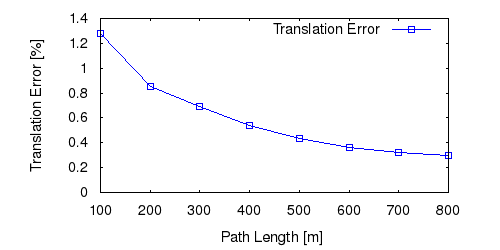This figure as: png eps pdf txt gnuplot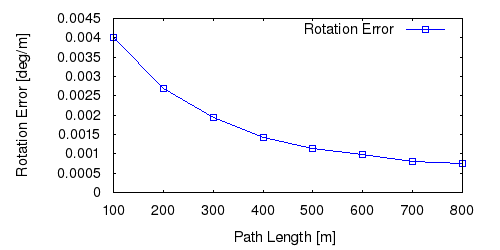This figure as: png eps pdf txt gnuplot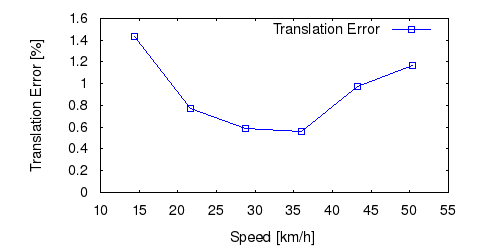This figure as: png eps pdf txt gnuplotThis figure as: png eps pdf txt gnuplot

## Sequence 14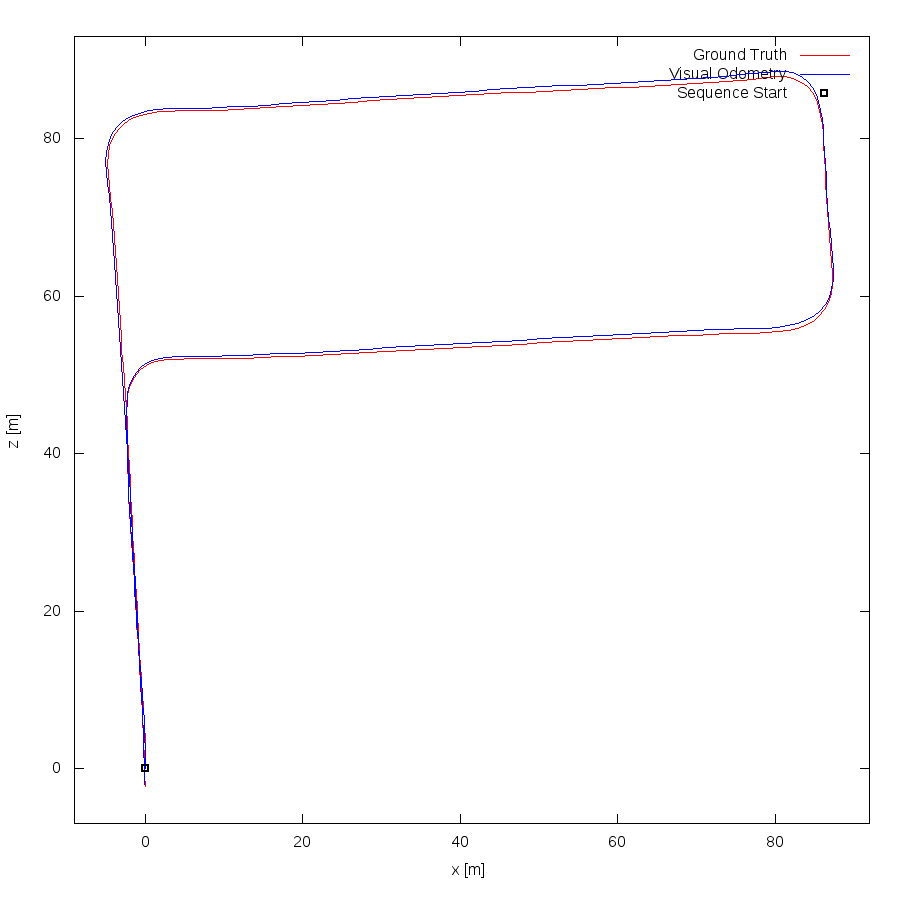This figure as: png eps pdf txt gnuplotThis figure as: png eps pdf txt gnuplotThis figure as: png eps pdf txt gnuplot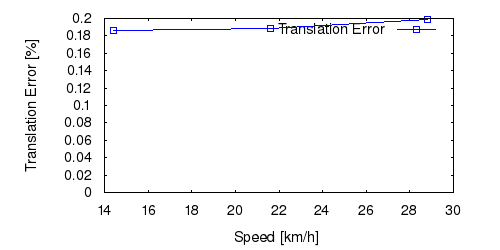This figure as: png eps pdf txt gnuplot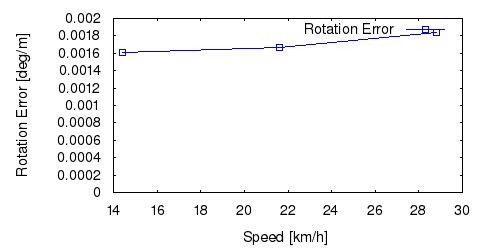This figure as: png eps pdf txt gnuplot

## Sequence 15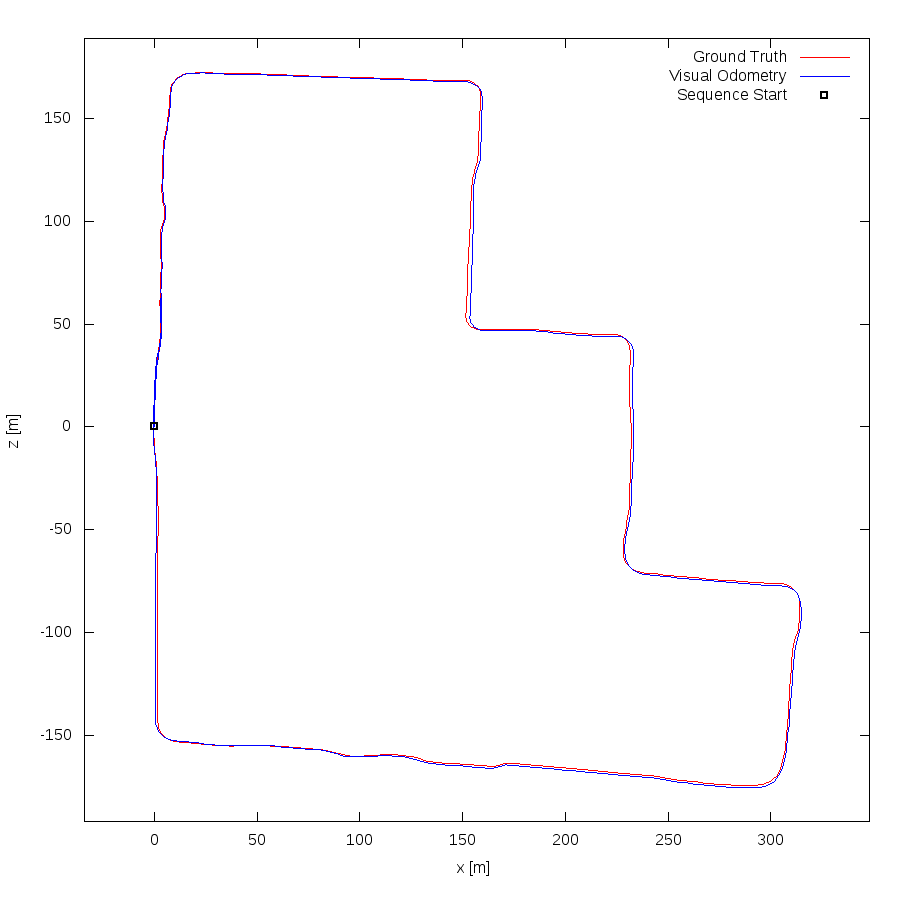This figure as: png eps pdf txt gnuplot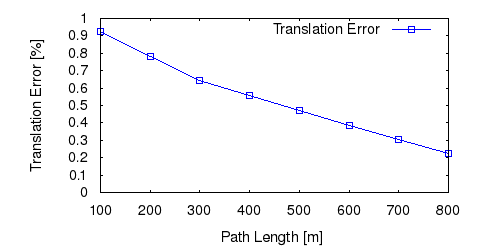This figure as: png eps pdf txt gnuplot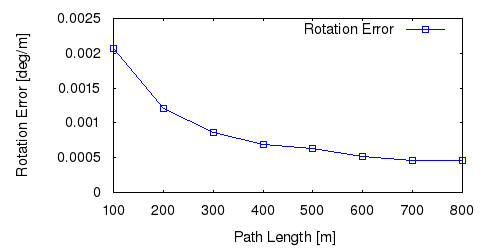This figure as: png eps pdf txt gnuplot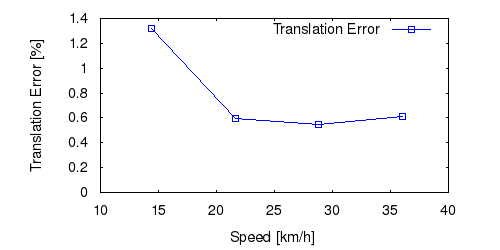This figure as: png eps pdf txt gnuplot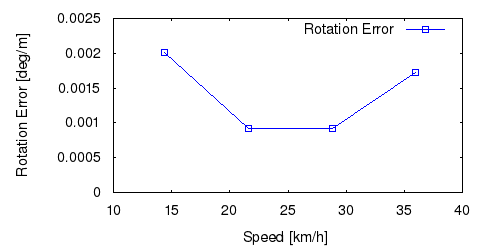This figure as: png eps pdf txt gnuplot